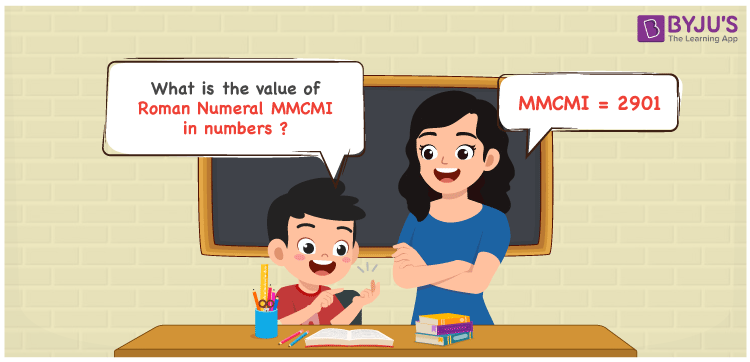Checkout JEE MAINS 2022 Question Paper Analysis : Checkout JEE MAINS 2022 Question Paper Analysis :

# MMCMI Roman Numerals

MMCMI Roman Numerals is 2901. In this article, the roman numerals MMCMI is split and the number indicating each English alphabet is mentioned. Using the addition concept, you will obtain the numeric form of the given Roman numerals. Students have to go through the Roman numerals articles on a daily basis to avoid last minute confusion during the exam preparation. Therefore, MMCMI can be indicated as 2901 in numbers.

 Number Roman Numeral 2901 MMCMI## How to Write MMCMI Roman Numerals in Numbers?

MMCMI is expanded and written in numbers in a clear cut format for the understanding of the students.

MMCMI = M + M + (M – C) + I

MMCMI = 1000 + 1000 + (1000 – 100) + 1

MMCMI = 2901

## Video Lesson on Roman Numerals## Frequently Asked Questions on MMCMI Roman Numerals

### Why is the roman numeral of 2901 written as MMCMI?

We know that

MMCMI = M + M + (M – C) + I

MMCMI = 1000 + 1000 + (1000 – 100) + 1

MMCMI = 2901

### Determine the value of 3000 – 99.

We know that

3000 – 99 = 2901

Hence, the value of 3000 – 99 is 2901 which is written as MMCMI.

### What is the remainder we obtain when MMCMI is divided by VI?

We know that

MMCMI = 2901

VI = 6

So the remainder we obtain when MMCMI is divided by VI is III.